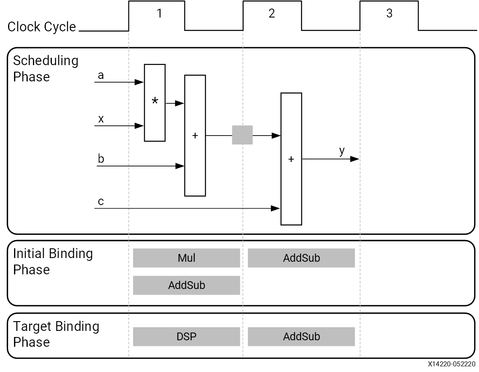# Scheduling and Binding Example - 2022.1 English

## Vitis High-Level Synthesis User Guide (UG1399)

Document ID
UG1399
Release Date
2022-06-07
Version
2022.1 English

The following figure shows an example of the scheduling and binding phases for this code example:

``````int foo(char x, char a, char b, char c) {
char y;
y = x*a+b+c;
return y;
}``````
Figure 1. Scheduling and Binding ExampleIn the scheduling phase of this example, high-level synthesis schedules the following operations to occur during each clock cycle:

• First clock cycle: Multiplication and the first addition
• Second clock cycle: Second addition, if the result of the first addition is available in the second clock cycle, and output generation
Note: In the preceding figure, the square between the first and second clock cycles indicates when an internal register stores a variable. In this example, high-level synthesis only requires that the output of the addition is registered across a clock cycle. The first cycle reads `x`, `a`, and `b` data ports. The second cycle reads data port `c` and generates output `y`.

In the final hardware implementation, high-level synthesis implements the arguments to the top-level function as input and output (I/O) ports. In this example, the arguments are simple data ports. Because each input variable is a `char` type, the input data ports are all 8-bits wide. The function `return` is a 32-bit `int` data type, and the output data port is 32-bits wide.

Important: The advantage of implementing the C code in the hardware is that all operations finish in a shorter number of clock cycles. In this example, the operations complete in only two clock cycles. In a central processing unit (CPU), even this simple code example takes more clock cycles to complete.

In the initial binding phase of this example, high-level synthesis implements the multiplier operation using a combinational multiplier (Mul) and implements both add operations using a combinational adder/subtractor (AddSub).

In the target binding phase, high-level synthesis implements both the multiplier and one of the addition operations using a DSP module resource. Some applications use many binary multipliers and accumulators that are best implemented in dedicated DSP resources. The DSP module is a computational block available in the FPGA architecture that provides the ideal balance of high-performance and efficient implementation.